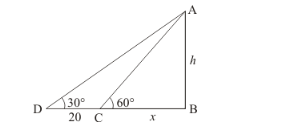# A T.V. Tower stands vertically on a bank of a river. From a point on the other bank directly

Question:

A T.V. Tower stands vertically on a bank of a river. From a point on the other bank directly opposite the tower, the angle of elevation of the top of the tower is 60°. From a point 20 m away this point on the same bank, the angle of elevation of the top of the tower is 30°. Find the height of the tower and the width of the river.

Solution:

Let AB be the T.V tower of height m on a bank of river and D be the point on the opposite of the river. An angle of elevation at top of tower is 60° and from a point 20m away then angle of elevation of tower at the same point is 30°. Let AB = h and BC = x.

Here we have to find height and width of river.

The corresponding figure is hereIn $\Delta C A B$

$\Rightarrow \quad \tan 60^{\circ}=\frac{A B}{B C}$

$\Rightarrow \quad \sqrt{3}=\frac{h}{x}$

$\Rightarrow \quad \sqrt{3} x=h$

$\Rightarrow \quad x=\frac{h}{\sqrt{3}}$

Again in $\triangle D B A$

$\Rightarrow \quad \tan 30^{\circ}=\frac{A B}{B C}$

$\Rightarrow \quad \frac{1}{\sqrt{3}}=\frac{h}{20+x}$

$\Rightarrow \quad \sqrt{3} h=20+x$

$\Rightarrow \quad \sqrt{3} h=20+\frac{h}{\sqrt{3}}$

$\Rightarrow \sqrt{3} h-\frac{h}{\sqrt{3}}=20$

$\Rightarrow \quad \frac{2 h}{\sqrt{3}}=20$

$\Rightarrow \quad h=10 \sqrt{3}$

$\Rightarrow \quad x=\frac{10 \sqrt{3}}{\sqrt{3}}$

$\Rightarrow \quad x=10$

Hence the height of the tower is $10 \sqrt{3} \mathrm{~m}$ and width of river is $10 \mathrm{~m}$.